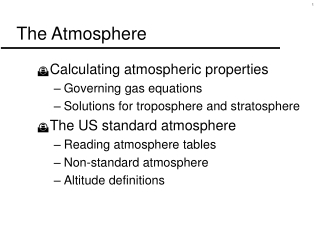DownloadDownload PresentationThe Atmosphere

# The Atmosphere

Download Presentation## The Atmosphere

- - - - - - - - - - - - - - - - - - - - - - - - - - - E N D - - - - - - - - - - - - - - - - - - - - - - - - - - -
##### Presentation Transcript

1. The Atmosphere • Calculating atmospheric properties • Governing gas equations • Solutions for troposphere and stratosphere • The US standard atmosphere • Reading atmosphere tables • Non-standard atmosphere • Altitude definitions

2. Governing Gas Equations • Equation of stateP = rRT • True for a “perfect gas” • Here, P= pressure [lb/ft2] = density [slug/ft3] R= gas constant = 1716 [ft lb/slug °R] T= temperature (absolute) [°R]

3. Governing Gas Equations • Hydrostatic equation dP = -rgdh • Ordinary differential equation • Geopotential altitude, h • Gravitational acceleration, g varies • below 50,000 ft, differences are small • assume geometric altitude = geopotential altitude

4. Calculating Atmospheric Properties • Mathematical relationships for properties in the atmosphere • Based on governing equations and temperature model • Needed for predicting aircraft / rocket performance at different altitudes • Solve the hydrostatic equation (an ordinary differential equation) • Temperature used as boundary conditions • Two regions of interest require two solutions

5. Troposphere Equations • Temperature gradient Ts= sea-level temperature hs= sea-level altitude = 0 ft dT/dh= lapse rate = -0.00356 °R/ft • Solve hydrostatic equation for pressure Ps= sea-level pressure = 2116.2 lb/ft2 • Use equation of state for density

6. Stratosphere Equations • Isothermal (to 82,000 ft) T36,152 = temperature at 36,152 ft • Solve hydrostatic equation for pressure P36,152= pressure at 36,152 ft = 472.7 lb/ft2 • Use equation of state for density

7. 1) Start with sea-level conditions 2) Check altitude 3) If in troposphere (h  36,152 ft) a) find T at h b) find P at h 4) If in stratosphere (36,152 ft < h < 82,000 ft) a) find T at 36,152 ft b) find P at 36,152 ft c) set T = T36,152 d) use T36,152 and P36,152 to find P at h 5) Find  from equation of state Calculating Atmospheric Properties

8. Calculating Atmospheric Properties Find temperature, pressure and density at 12,000 ft 1) Start with sea-level conditions Ts = 518.69°R (about 59°F) Ps = 2116 lb/ft2 rs = 0.002377 sl/ft3 2) Check altitude (which atmospheric region?) 3) a) Find T at h

9. Calculating Atmospheric Properties 3) b) Find P at h 4) Calculate r

10. The US Standard Atmosphere • Based on mean annual US temperature • Uses empirical temperature data • Reference of atmospheric conditions • Appendix B, Introduction to Aeronautics • Table B.1 English (USCS) units, Table B.2 SI units • Includes speed of sound, a and viscosity, m